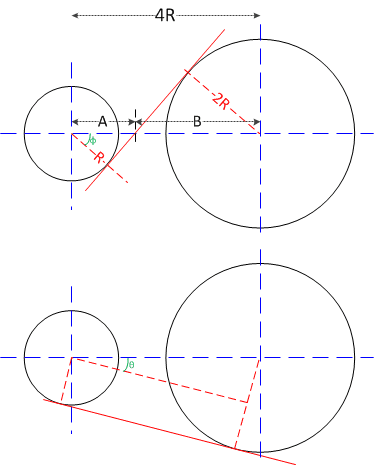# Probability of hitting a rotating disc

Homework Statement:
There are two discks rotating with angular velocity w and with radius R and 2R. The distance from there centres is 4R. Find the probability of a particle, that was rotating on the board of the smaller disck, to shock with the bigger after it leaves de disck.
Relevant Equations:
V=wr , P(E)=N(E)/U
There are two points of the smaller disck such that a line is tangent to the two discks and passes by that point. If the particle leaves of any point belongin to the arch that connects these two points, then it will hit the other disck. So I managed to calculate the value of this arch and divide it by 2π. But I got the wrong answer. Is this correct ?

#### Attachments

•15652743478657201379945105609649.jpg
32.3 KB · Views: 61

BvU
Homework Helper

If you don't post what you did, no further help is possible. Show your work ! In detail.

 by the way, is this happening in the vertical plane ? Or did you just rotate the picture to annoy us ?

Last edited:
haruspex
Homework Helper
Gold Member
2020 Award
There are two points of the smaller disck such that a line is tangent to the two discks and passes by that point. If the particle leaves of any point belongin to the arch that connects these two points, then it will hit the other disck.
So far so good, but what value did you calculate for the arc length?

•Moara
If you statement is: "it's the wrong answer", then I suppose your statement is correct.

If you don't post what you did, no further help is possible. Show your work ! In detail.

 by the way, is this happening in the vertical plane ? Or did you just rotate the picture to annoy us ?
Well,
If you statement is: "it's the wrong answer", then I suppose your statement is correct.

If you don't post what you did, no further help is possible. Show your work ! In detail.

 by the way, is this happening in the vertical plane ? Or did you just rotate the picture to annoy us ?
The movement is in the horizontal plane, sorry about the image.

BvU
Homework HelperI understood that -- I was just being a bit ironic.

Now: both Haru and I are asking you to show your work -- can you do that ?

BvU
Homework Helper
•Moara
Found my mathematical error while writing my attempt, thank you

•BvU
BvU
Homework Helper
Often happens -- yet another reason we require posting of a solution attemptBvU
Just so you know we take your problems seriously( I did however use the wrong sign for ##\omega##)
•